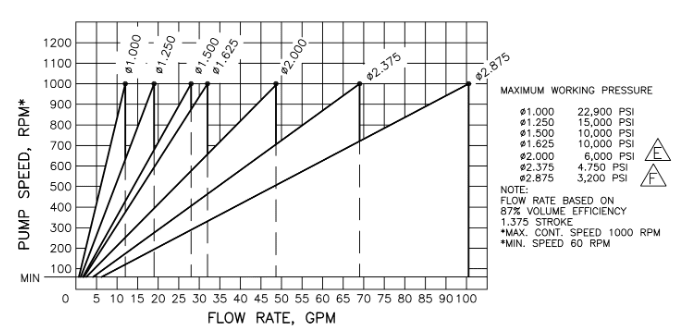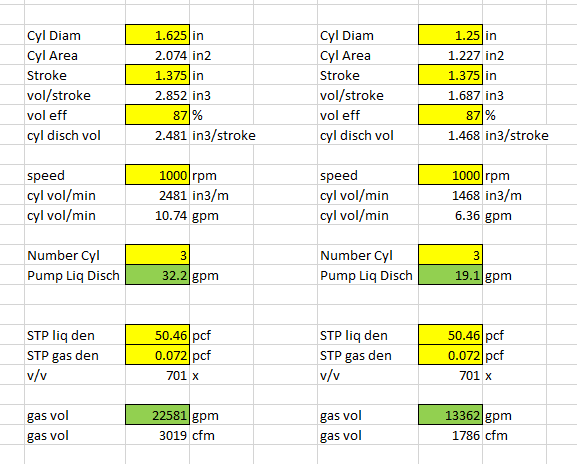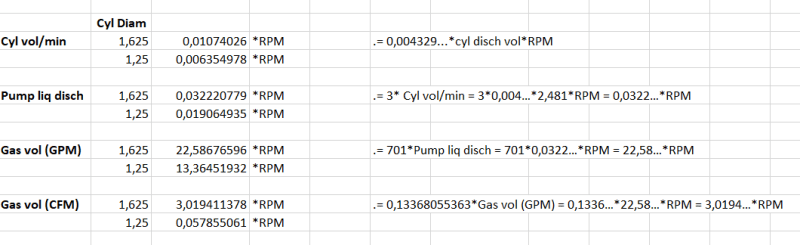×
INTELLIGENT WORK FORUMS
FOR ENGINEERING PROFESSIONALS

Are you an
Engineering professional?
Join Eng-Tips Forums!
• Talk With Other Members
• Be Notified Of Responses
• Keyword Search
Favorite Forums
• Automated Signatures
• Best Of All, It's Free!

*Eng-Tips's functionality depends on members receiving e-mail. By joining you are opting in to receive e-mail.

#### Posting Guidelines

Promoting, selling, recruiting, coursework and thesis posting is forbidden.

# Calculating volumetric flow rate without rotameter

## Calculating volumetric flow rate without rotameter

(OP)
Hi,

I'm wondering how You can calculate volumetric flow rate (SCFM=ft2/min) without rotameter installed.

We need to change from 1,625" to 1,25" Cold End. This will change the volumetric flow rate.

Liquid nitrogen is pumped by a hydraulic pump to a vaporizor. How can I calculate gasses volumetric flow rate out of vaporizor?

### RE: Calculating volumetric flow rate without rotameter

How you doing this Pump?
Pump rate?
Free flow rate?
What do you know? Anything?

If you know the mass rate, you can figure volume like this.

### RE: Calculating volumetric flow rate without rotameter

(OP)
General Specs of the Pump:

Pump rotation: Bi-directional
Stroke: 1,375"
Max pump speed: 1200 RPM
Max power output: 200HP
Cold End bore size: 1,25"
Max discharge pressure: 15000psi

On the datasheet for vaporizor used, it states that:
- Gas ρ = 0,072 lb/ft3
- Specific volume = 13,8889 ft3/lb
- Vapor density = 0,967 and Liquid density@BP: 50,46 lb/ft3

I also read that liquid to gass expansion ratio of nitrogen is 1:694 at 20deg C.

The Bernoulli's equation is: P1+1/2ρv1^2+ρgh1=P2+1/2ρv2^2+ρgh2

Known data:
P1 = Pressure out of hydraulic pump
P2 = Pressure of gas coming out of vaporizor (backpressure)
ρ = density of fluid
g, h1 and h2

As I want to solve for v2, which is the velocity of the medium going out of vaporizor, I need one more input, v1. How can I obtain velocity of the fluid going in to the vaporizor?

Also, can I really use Bernoulli's equation if the density of fluid going in is different than density of gass coming out of vaporizor?

If my understanding is correct, having found v2, I can use v2 = q2/A = 4q/(D^2*π) or
q2 = (v2*D^2*π)/4 to find volumetric flow rate (q2). However, this equation only stands for flat, plane cross-sections. Otherwise, it's q = vAcosθ. Where θ is the angle between the unit normal n̂ and the velocity vector v of the substance elements.

Cheers and thank You!

### RE: Calculating volumetric flow rate without rotameter

(OP)
Hi again,

I found out that the value I'm looking for is not exactly the volumetric flow rate...The value I want to find is SCFM (Standard Cubic Feet Minute), which is not the same as volumetric flow rate, as I first thought.

SCFM is not dependant on either temperature or pressure, as it takes standard values for these. (P=1atm, T=68°F).
My former collegue came with the strange assumption that SCFM = 3*RPM of the pump. How can that be?

### RE: Calculating volumetric flow rate without rotameter

SCFM = 3*RPM sounds strange for me. Did he tell you how he had come up with this idea?
I would like to discover this one :)

### RE: Calculating volumetric flow rate without rotameter

(OP)

#### Quote (Fudji)

SCFM = 3*RPM sounds strange for me. Did he tell you how he had come up with this idea?
I would like to discover this one :)

So it does for me. I have no idea how he came up with this and he is no longer working here. I'll try to contact him and see what he responds. In the meantime I know that one can obtain CMF with this equation:

CMF = (CID*RPM*VE)/3456, where:
CID: Engines displacement(in^3)
VE: volumetric efficiency (VE=3 for engines with turbo, maybe he took value 3 from here?)

Even if ... SCFM = CFM*(Pactual/14,7psi)*(68°F/Tactual) and he is taking the RPM from the hyrdraulic pump in his formula.

### RE: Calculating volumetric flow rate without rotameter

If you know the volume of gas at any pressure and temperature, you can find the volume at any other pressure and temperature.

P1 x V1 / T1 = P2 x v2 / T2
Solve for the value you want.
Pressures and temperatures are absolute values and compressibility is neglected.
Absolute temperature is °Rankin "R" degrees = F + 459.67
Compressibility will be important at high pressures. It is also gas dependent.

Pump displacement per minute is = Cylinder volume x rpm
If a piston pump doing gas compression, there is an initial volume of gas taken in when stroke is at 0 position. That amount of gas or liquid in the cylinder depends on the inlet pressure and temperature. The volume at the outlet depends on the discharge pressure.
When at full stroke, there is some additional length of the cylinder where the N2 is compressed to its new volume V2. Calculate that and you can find the pressure.
How have to estimate the temperature using the gas k value.

A liquid pump's capacity is cylinder displacement/stroke x rpm. Discharge pressure will be whatever pressure is needed for the liquid to get into the downstream piping. That's controlled by how you are operating that equipment and how that affects pressure at the pump discharge.

The data sheet values are for N2 at Standard conditions. N2 weighs 0.072 lbs/ft3 at STP.
The inverse of that is specific volume 13.8ft3, the volume needed to have a pound of air at STP. I guess the liquid N2 density is 50.46lbs/ft3

### RE: Calculating volumetric flow rate without rotameter

(OP)

#### Quote (1503-44)

Thank You for the explanation.

I can't operate with numbers. I have to come up with the equation that gives SCFM as an output.
The client wants to change cold ends from 1,625" to 1,25" on 901-01966 ICP-200 pump.
It seemed logical to me that this will change SCFM output. However, the equation my former collegue used was 3xRPM and this would imply that SCFM is independent of both pressure and temperature.

From the data chart of the pump, flow rate:pump speed is a linear function. We can write Flow Rate (GPM), as a function of RPM.

For Ø1.625 Cold Ends: Flow Rate (GPM) = 0,032*RPM
For Ø1.250 Cold Ends: Flow Rate (GPM) = 0,019*RPMVAPORIZER SPECIFICATIONS

Capacity: 180,000 SCFH
MAWP N₂: 15,000 PSI
MAWP H₂O: 150 PSI
H₂O Flow Required: 120-140 GPM
H₂O Temperature Required: 185° F
H₂O ∆T @ 120 GPM: 45° F
H₂O ∆T @ 140 GPM: 35° F
H₂O ∆P @ 140 GPM: 25-35 PSI
N₂ ∆P @ 180K & 10K PSI: 200 PSI

### RE: Calculating volumetric flow rate without rotameter

Weight of liquid in must equal the weight of gas out.

#### Quote:

Liquid nitrogen is pumped by a hydraulic pump to a vaporizor.

#### Quote:

I also read that liquid to gass expansion ratio of nitrogen is 1:694 at 20deg C.
If the liquid temperature is adjusted for 20deg C, then each stroke or revolution will deliver a known weight of nitrogen.
My WAG is that your colleague used the volume of one pump RPM to determine the weight of nitrogen per revolution.
He then calculated that That volume of liquid times 694 times 3 equalled 1 cu ft at SCF conditions.
I don't know what you mean by the "cold end". But, if the cold end change does not change the volume per revolution there should be no change in the weight per revolution.
If the cold end is a restriction, then the velocity of the gas will change but not the SCFM.
Weight in equals Weight out.
If the cold end change does directly change the pump displacement per stroke, then the change in SCFM will be in the ratio of 1.252 / 1.6252 = 0.592

--------------------
Ohm's law
Not just a good idea;
It's the LAW!

### RE: Calculating volumetric flow rate without rotameter

Yeah it does. The cold end size is the piston diameter as you have guessed.

There are 3 cylinders, right?

Pump discharge = cylinder volume x number of cylinders x rpm x volumetric efficiency
cylinder volume is 1/4 pi D^2 x stroke
volumetric efficiency 0.87 per your pump chart. A volumetric efficiency 0f 0.87 indicates that the stroke does not empty the cylinder completely. There will be 13% of the cylinder volume that will remain in the cylinder.

The pump will give you the calculated discharge up to the max pressures as per your chart. The pump apparently runs out of sufficient power for each cold end diameter at the pressures indicated.DON'T USE THIS SPREADSHEET ATTACHED HERE.
GAS VOLUME IS GPM, NOT CFM.
I CORRECTED THE PICTURE, BUT CAN'T SEEM TO CORRECT THIS SPREADSHEET LINK.
THE CORRECTED SHEET FOLLOWS.

### RE: Calculating volumetric flow rate without rotameter

(OP)

#### Quote (1503-44)

The other one has the wrong gas volume as cfm, but it is gpm
This one gives both like the picture above.
Gas volume is standard US gallons and standard cubic feet.

Good morning!
Wow, I did not expect that someone will give so much help to a stranger on the internet. Thank You so much! I really appreciate Your time spent on this. Any way I can give back to You? (tipjar, anything?)

After going through the spreadsheet, I now understand how the calculations should have been made.
Defining Gas vol(cfm) in terms of RPM should then be:

3,0194...*RPM for 1,625 cylinder diameter
1,7865...*RPM for 1,25 cylinder diameterThis proves that assumption 3*RPM that my collegue made was correct and that the value of SFCM is changed with piston diameter.
Thank You again!

### RE: Calculating volumetric flow rate without rotameter

You are welcome. Glad you understand how to do it now.

### RE: Calculating volumetric flow rate without rotameter

(OP)
I now went a little bit further and developed an equation for "gas volume per minute" for any given piston diameter for this case scenario.

Vcmf(D,RPM) = 1,1434241355938155786971828180212*D2*RPM = 1,1434*D2*RPM

where D is piston diameter in inches.

### RE: Calculating volumetric flow rate without rotameter

Too many significant figures exceed the margin of error of the cylinder diameter's machining tolerance.### RE: Calculating volumetric flow rate without rotameter

(OP)
That is true, didn't think of this.

I should probably stick with 1,14*D2*RPM

### RE: Calculating volumetric flow rate without rotameter

Yeah. Too many numbers makes my head spin around.. like in the Exorcist.

#### Red Flag This Post

Please let us know here why this post is inappropriate. Reasons such as off-topic, duplicates, flames, illegal, vulgar, or students posting their homework.

#### Red Flag Submitted

Thank you for helping keep Eng-Tips Forums free from inappropriate posts.
The Eng-Tips staff will check this out and take appropriate action.

#### Resources

Low-Volume Rapid Injection Molding With 3D Printed Molds
Learn methods and guidelines for using stereolithography (SLA) 3D printed molds in the injection molding process to lower costs and lead time. Discover how this hybrid manufacturing process enables on-demand mold fabrication to quickly produce small batches of thermoplastic parts. Download Now
Examine how the principles of DfAM upend many of the long-standing rules around manufacturability - allowing engineers and designers to place a partâ€™s function at the center of their design considerations. Download Now
Industry Perspective: Education and Metal 3D Printing
Metal 3D printing has rapidly emerged as a key technology in modern design and manufacturing, so itâ€™s critical educational institutions include it in their curricula to avoid leaving students at a disadvantage as they enter the workforce. Download Now

Close Box

# Join Eng-Tips® Today!

Join your peers on the Internet's largest technical engineering professional community.
It's easy to join and it's free.

Here's Why Members Love Eng-Tips Forums:

•Talk To Other Members
• Notification Of Responses To Questions
• Favorite Forums One Click Access
• Keyword Search Of All Posts, And More...

Register now while it's still free!